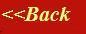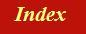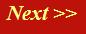#### Tutorials:

TEM alignment

STEM alignment

Wave interference

#### Research:

Diffractive imaginging

## The Fourier transform of a one-dimensional aperture (a harbour entrance)

You can find what we derive in this page in any textbook on optics or Fourier transforms. What you probably wonĺt find is the geometrical way Iĺm going to derive the result, using my analogy of phase threads and the mechanical Fourier transform machine. The result we obtain is exceedingly important in the wave optics and electron microscopy, for all sorts of reasons, even though it may seem at first rather uninteresting.

What we are going to work out is the amplitude distribution of a plane wave that has passed through a harbour entrance when is arrives in the far- field Fraunhofer diffraction plane. That is, we want to work out the Fourier transform of the Ĺtop hatĺ function:This function is zero everywhere except between x =-a and x=a, where it has a real value of A. In other words, it is a harbour wall opening of width 2a with a plane wave incident upon of modulus A. Because there are no imaginary parts of the wave, its phase is zero throughout the whole harbour wall aperture. By putting the function symmetrically in the middle of the x-axis, then all our maths is much easier (the Fourier transform is entirely real ľ any symmetric real function has a Fourier transform which is entirely real).

Now, in terms our phase thread analogy, I suggested we could calculate a Fourier transform using a machine that looked like this: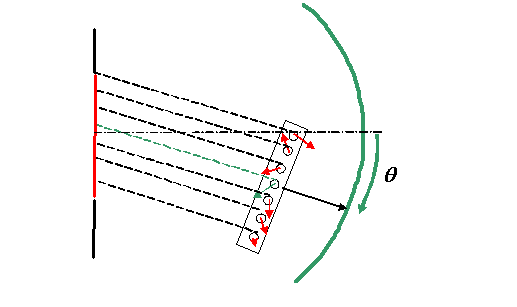(see previous page)

As the machine rotates around the green Gaussian surface (which in practice is very long way from the harbour entrance), the phase threads pull the little wave value vectors round and round in the complex plane, via the pulleys, each of which has a circumference of the radiation wavelength. The Fourier transform (the amplitude of the Fraunhofer diffraction pattern) is the vector addition of all these little wave values.

Well, in the case of our empty one-dimensional aperture (the harbour wall gap), this process is quite easy to picture. When θ=0, we have to add up a whole load of little vectors, all of which are real and of the same length. Iĺm going to draw the addition of all these vectors like this:The green vector is the wave value emanating from the centre of the aperture (at x=0): at all values of θ, this vector stays purely real (pointing to the right), because no phase difference is introduced to it as we move the mechanical Fourier transform device around the Gaussian surface.

At some small value of θ all the red vectors rotate by a small amount. We add them together as before, tail to head, and so this Ĺsnakeĺ of little vectors begins to wrap itself up, like this:Remember ľ the green vector stays purely real (pointing to the right along the real axis of the complex plane). Vectors further away from the green vector change in phase quicker (as a function of θ) than those close to it, because the path length change in their phase threads is proportional to their distance from x=0. You see now why it is convenient to define the position of the aperture centred on x=0, because by symmetry the black resultant vector (which is the value of the Fourier transform at a particular θ) is horizontal ľ it is always real. (If we chose one of the other vectors to be always real ľ in other words we shifted the aperture to one side, then the resultant vector would be the same length, but it would also rotate in phase: this is the so- called Ĺshift theoremĺ in Fourier-transform-theory- speak.)

Okay. Now if you can picture how the resultant (black) vector changes as the Ĺsnakeĺ of the red vectors gets more and more tightly wound around, then you really can do a Fourier transform in your head!

Thinking about it, the red vectors form part of the arc of a circle. This total arc length remains constant at all values of θ, but the radius of the circle gets smaller and smaller as θ increases.

Lets just draw the sum of red vectors as a red line (drawing lots of tiny red pointers is deadly dull). The next diagram shows what happens to the red line and the resultant black vector as θ increases:By the time we have reached the penultimate diagram, the red circle has wrapped right over itself, and so the resultant vector has changed sign. At higher and higher values of θ the circle becomes even tighter, wrapping around on itself over and over again, getting smaller and smaller, with the resultant vector repeatedly changing sign.

If we plot the resultant vector as a function of θ this is what we can guess it will look like:And indeed, this is exactly the Fourier transform of the top-hat function. Because it crops up all over the place in optics is has a special name: the sinc function.

As a normal mortal electron microscopist, all you really need to know about this function is its shape, the fact as the aperture gets wider (Ĺaĺ in the first diagram gets larger) then the sinc function gets proportionally narrower. (Such a simple fact can be used to give a kinematical explanation of thickness fringes we see in diffraction contrast.)

You can derive the formal mathematical expression for this function easily by just doing the Fourier transform: this is done in hundreds of textbooks. Here, Iĺm going derive the same solution by using a geometric construction from the folding-up circle.

### The sinc function as a writhing snake:

The Ĺwrithing snakeĺ refers to the red circle above, which slowly ties itself into a tight knot. What I want to do is to work out the scattering amplitude (the length of the black resultant vector in the diagrams above) as a function of scattering angle, θ, using just simple geometry.

The first thing to worry about is the total length of the snake (the red line, made up of lots of little complex vectors). Well, we worked out before that at θ= 0, the Fourier transform is just the integral of the whole function we are transforming. In our case, because all the vectors are parallel (zero phase) that means the entire length of snake is just 2aA.

Next, I want to worry about the radius of the circle made by the writhing snake. To work this out, lets zoom into a bit of the writhing snake, like this:Here we are pretending the snake is made of discrete wave amplitude vectors. In fact, we can let these tend to having zero length, but it is easier to start like this. Now Δφ is the phase angle introduced by phase threads attached to adjacent bits of the wave function, separated by a small distance, δx, like in the next diagram.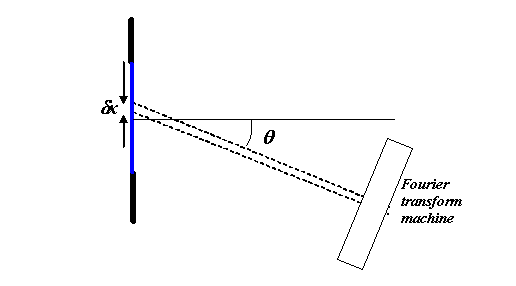where have drawn our Fourier transform machine (see previous page). Clearly, Δφ is 2π p/λ, where p = sinθ, but because as usual we are only interested in small θ, then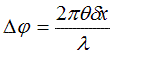To make life simpler, Iĺm going get rid of all the constants by putting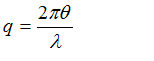so that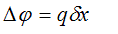Going back to our red vectors, we have to find out the length of each incremental bit of the snake. If the total length of the snake is 2aA when x=2a, then the length of the amplitude of wave corresponding to the elemental bit δx is Aδx. We can use this to work out the radius of circle of the writhing snake. For very small δx, we can see from the following diagram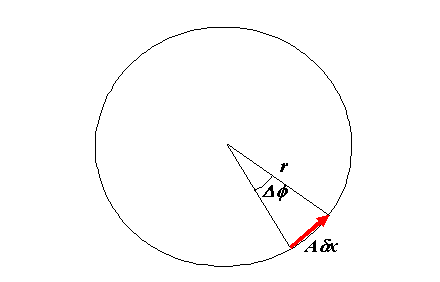that the radius, r, is given by r=Aδx/Δφ. So, substituting for Δφ, we haveNow we know the radius of the circle and the length of the snake, itĺs easy to work out the resultant amplitude, which Iĺll call F. Look at the next diagram:The resultant black vector is of length 2r sinα, and 2α is just the length of blue arc divided by r. The length of the blue arc is just the circumference of the circle (2πr) minus the length of the whole snake, 2aA. That is to say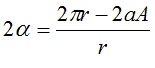or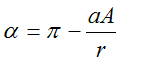So, finally we can write:If we remember our elementary trig (I never can, but if you sketch a sine curve you can work it out), then we know that sin (π-aq)=sin(aq), soIĺve multiplied top and bottom by Ĺaĺ, to emphasise that sin aq is divided by aq. In the textbooks, youĺll see this written with a further substitution, such thatgiving a final result (remembering our definition of q), that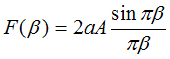For a top hat function of unity height and width, this reduces to sinπβ/πβ, which is the formal definition of a sinc function. The sinc is just a sine wave divided 1/(angle in the sine). The scaling of β by π means that the function is zero whenever β is an integer.

We can use the sinc function as a definition of the delta function, which in turn can make the mathematical manipulation of Fourier transforms, convolutions and their inverses, very easy. The sinc function is important in the scattering of waves from thin crystals (as often encountered in electron microscopy), and crops up in all sorts of other areas of space- and time-domain analysis, in both optics, quantum mechanics, solid state physics and even such fields as voice recognition.